Ch 1. Limits Multimedia Engineering Math Limit of aSequence Limit of aFunction LimitLaws Continuity RateChange
 Chapter 1. Limits 2. Derivatives I 3. Derivatives II 4. Mean Value 5. Curve Sketching 6. Integrals 7. Inverse Functions 8. Integration Tech. 9. Integrate App. 10. Parametric Eqs. 11. Polar Coord. 12. Series Appendix Basic Math Units Search eBooks Dynamics Fluids Math Mechanics Statics Thermodynamics Author(s): Hengzhong Wen Chean Chin Ngo Meirong Huang Kurt Gramoll ©Kurt GramollMATHEMATICS - CASE STUDY SOLUTION In a series cutting experiments, Jimmy summarized the relationship between force to cut the material and the heat released from the material. The heat is a piecewise continuous function of force. He wants to analysis the heat released when force is 1 and 2 kN. The function is: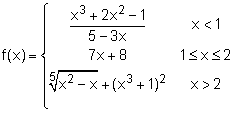Since f(x) is a piecewise function, the functions for f(x) on the left and right hand side of 1 kN and 2 kN. The way to analysis the boundary heat conductive is to consider both side heat conductive at the boundary. First, consider the case of x approaches 1-: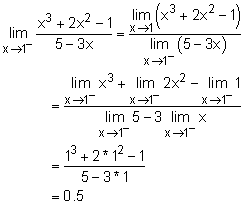Second, consider the case of x approaches 1+: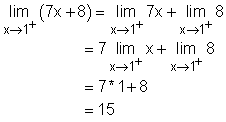Third, consider the case of x approaches 2-: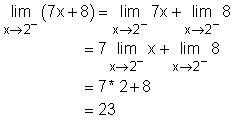Finally, consider the case of x approaches 2+: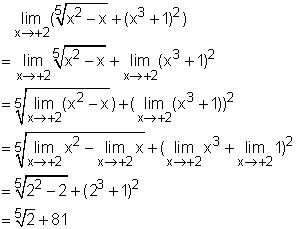Discussion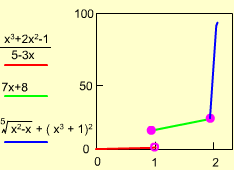Piecewise f(x) function diagram According to left and right hand limit,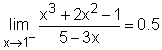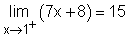In this situation, although both left and right hand limit exist, the limit at 1 kN does not exist. The heat conductive function is not a continuous function at this point. The function has a jump. The same situation happens at 2 kN. In order to solve the limit of a function, many limit laws can be applied. The proper application of the limit law can make the problem solving process easier. Of course, it does take practice.